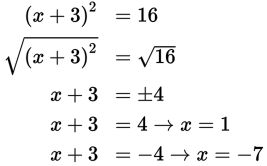# SAT Math Multiple Choice Question 588: Answer and Explanation

### Test Information

Question: 588

3. Which of the following are solutions to the quadratic equation (x + 3)2 = 16?

• A. x = –19 and x = 13
• B. x = –7 and x = 1
• C. x = –1 and x = 1
• D. x = –1 and x = 7

Explanation:

B

Difficulty: Medium